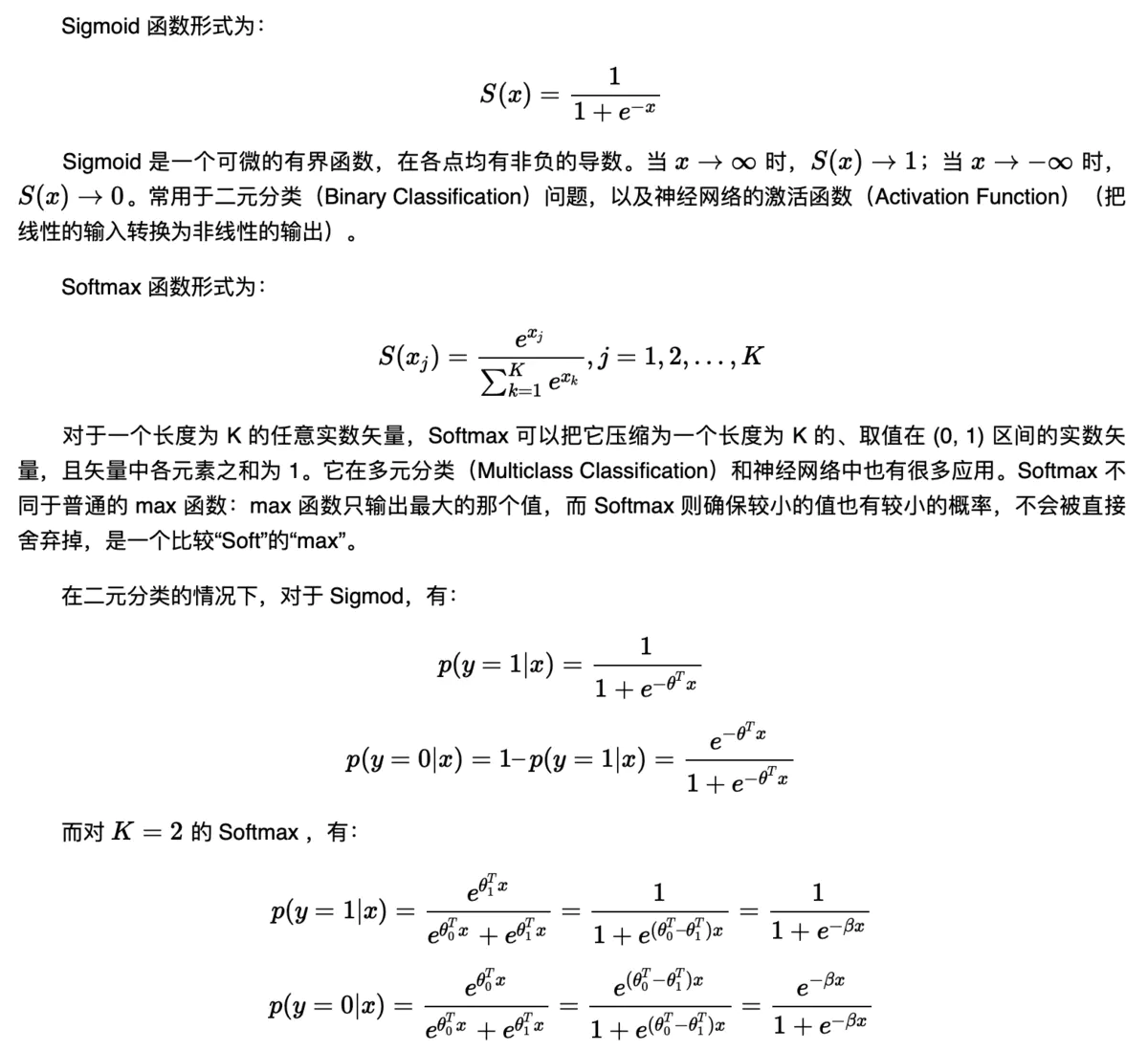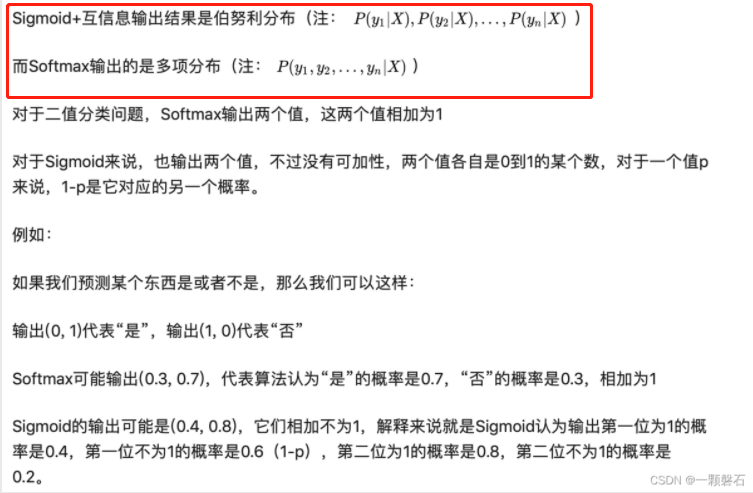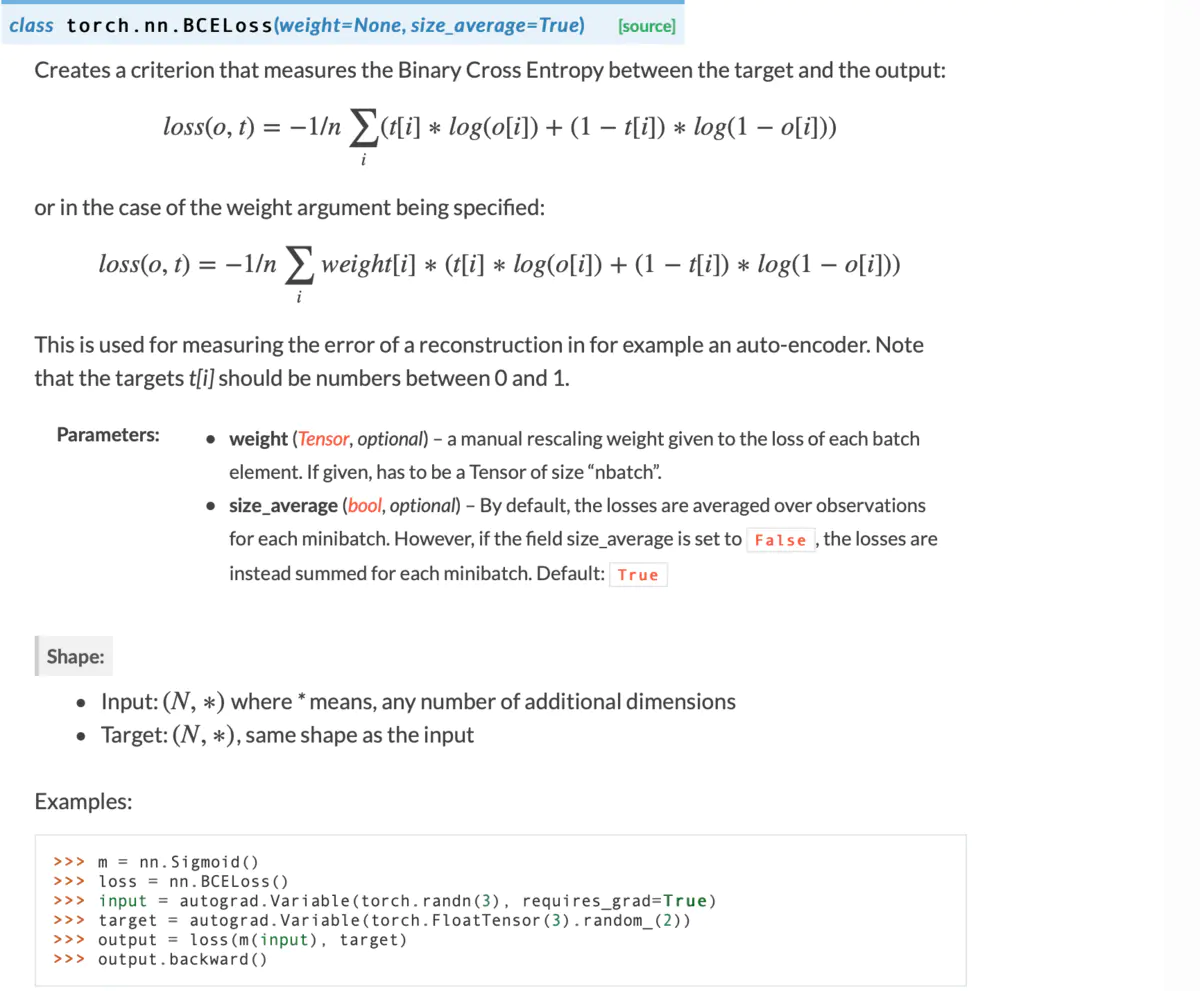#### 图像二分类问题—>多标签分类

L O S S = − ( y l o g ( p ( x ) + ( 1 − y ) l o g ( 1 − p ( x ) ) LOSS=-(ylog(p(x)+(1-y)log(1-p(x))

#### Sigmoid和Softmax的本质及其相应的损失函数和任务#### 多标签分类任务的损失函数BCE

(0, 0)图中既没人，也没手机
(0, 1)图中没人，但是有手机
(1, 0)图中有人，但是没手机
(1, 1)图中既有人，也有手机#### Pytorch的BCE代码和示例

>>> import torch
>>> output = torch.randn(3,3)
>>> output
tensor([[-0.8858,  0.3241,  0.9456],
[ 1.4887,  1.8076, -0.0565],
[-1.6529, -1.8539,  0.6756]])


>>> active_func = nn.Sigmoid()
>>> output = active_func(output)
>>> output
tensor([[0.2920, 0.5803, 0.7202],
[0.8159, 0.8591, 0.4859],
[0.1607, 0.1354, 0.6627]])


>>> target = torch.FloatTensor([[0,1,1],[1,1,1],[0,0,0]])
>>> target
tensor([[0., 1., 1.],
[1., 1., 1.],
[0., 0., 0.]])


>>> loss = nn.BCELoss()
>>> loss = loss(output, target)
>>> loss
tensor(0.4114)


#### 总结